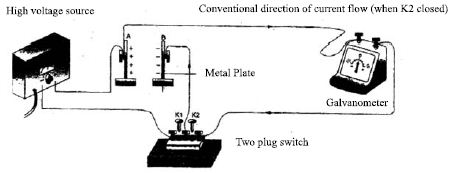# What is Electric Current?

When two bodies of different potential are connected by a conducting wire, electrons flow from the body of low potential to that of higher potential. This flow of electron continues until the potential difference between the two bodies becomes zero. If by any process the potential difference between the two objects is maintained, then this flow of electron goes on continuously. This continuous flow of electrons is electric current.

The amount of charge that flows in unit time through any cross section of a conductor is called electric current. If through any cross section of a conductor, the quantity of charge Q flows in time t, then the electric current will be I = Q/tThe unit of electric current is ampere. If an amount of charge 1 C flows in 1 second through any cross section of a conductor, then the quantity of electric current produced is called 1 A.